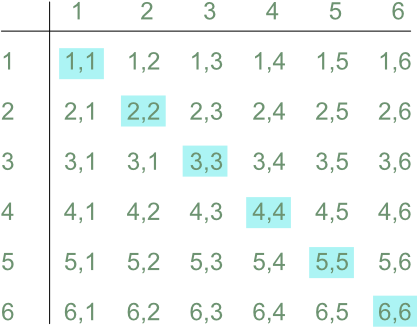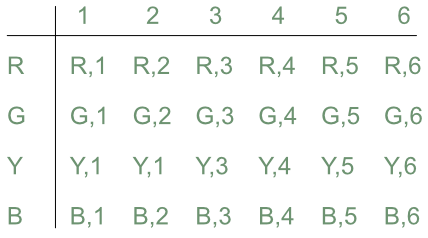Possibility Spaces

# Possibility Spaces

GCSE(F),

A Probability Space Diagram, or Sample Space Diagram, shows information about event outcomes in a structured view. Space diagrams are normally used to show the possible combination of two events.

The information held in the grid depends upon the events being recorded. A combination of a coin flip and a dice roll would show the face of the coin and the value of the dice roll for each entry. If two dice were being rolled, then each entry may be the value of the two dice added together.

Space diagrams are used to show theoretical events.

## Examples

1. Two dice are rolled. Using a space diagram, calculate the probability of rolling a double (that is, where both dice show the same value).

Answer: frac(1)(6)

There are 6 possible outcomes of doubles out of 36 possible throws = frac(6)(36) = frac(1)(6)

Space diagram showing combinations:2. A spinner with 4 possible outcomes and a normal 6-sided dice are used in a board game. The spinner can show either Red, Green, Blue or Yellow. Show, in a space diagram, the possible combinations for a spin of the spinner and a throw of the dice.

Answer: